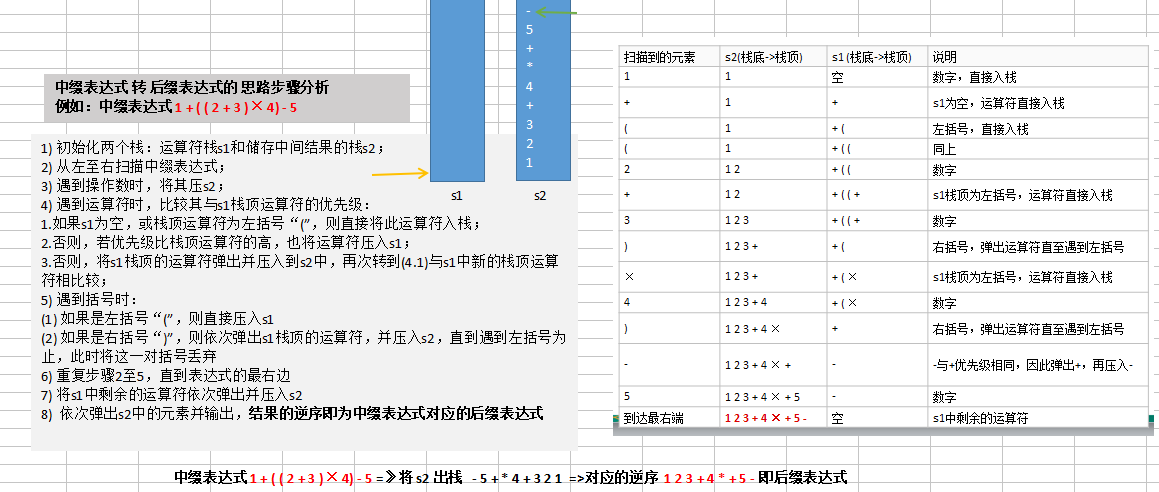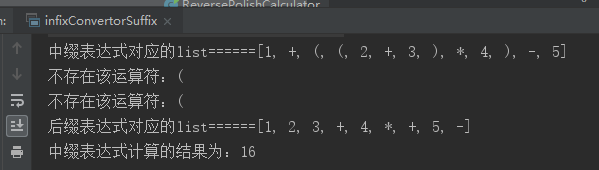• #include<stdio.h> #include<stdlib.h> #include<math.h> #define MAX 100 /* 表达式最大长度 */ #define true 1 #define false 0 /* 定义数据栈 */ typedef struct LinkStack1 { float data struct LinkStack1 *...
• 中缀表达式转为后缀表达式是实现逆波兰计算器的核心算法，其使用栈(stack) 这一数据结构实现。本文转化算法（java版本）支持 整数和括号的计算表达式转换。

中缀表达式转为后缀表达式 是实现逆波兰计算器的核心算法，其使用 栈(stack) 这一数据结构实现。本文转化算法（java版本）支持 整数和括号 的四则运算表达式转换。

学习方法:先快速了解定义，阅读一遍算法伪码。然后重点根据例子走一遍计算过程（结合伪码），最后看算法的具体实现。
目录1 前、中、后缀表达式2 中缀转后缀表达式算法2.1 转化算法伪码（由波兰数学家提出）2.2 具体示例 1+((2+3)×4)-5 转换步骤2.3 Java算法代码

1 前、中、后缀表达式
前缀表达式、中缀表达式、后缀表达式都是四则运算的表达方式,用于四则运算表达式求值。

中缀表达式：就是平常我们写的表达式。如1-(2+3)  和  1+((2+3)*4)-5。
前缀表达式（波兰式)：通俗来说就是运算符写在前面，操作数写在后面。如 1+((2+3)*4)-5 对应的前缀表达式为  - + 1  *  + 2 3 4 5  中缀转前缀也有一套算法。(前缀表达式介绍)
后缀表示式(逆波兰式）：运算符位于操作数之后，如 1+((2+3)*4)-5 对应的后缀表达式为 1 2 3 + 4 × + 5 -   本文重点介绍转化算法。

2 中缀转后缀表达式算法
2.1 转化算法伪码（由波兰数学家提出）

初始化两个栈：运算符栈s1和储存中间结果的栈s2；
从左至右扫描中缀表达式；
遇到操作数时，将其压s2；
遇到运算符时，比较其与s1栈顶运算符的优先级：

如果s1为空，或栈顶运算符为左括号“(”，则直接将此运算符入栈；
否则，若优先级比栈顶运算符的高，也将运算符压入s1；
否则，将s1栈顶的运算符弹出并压入到s2中，再次转到(4.1)与s1中新的栈顶运算符相比较；

遇到括号时：

如果是左括号“(”，则直接压入s1；
如果是右括号“)”，则依次弹出s1栈顶的运算符，并压入s2，直到遇到左括号为止，此时将这一对括号丢弃；

重复步骤2至5，直到表达式的最右边；
将s1中剩余的运算符依次弹出并压入s2；
依次弹出s2中的元素并输出，结果的逆序即为中缀表达式对应的后缀表达式。

2.2 具体示例 1+((2+3)×4)-5 转换步骤

扫描到的元素
s2 (栈底-栈顶)
s1 (栈底->栈顶)
说明

1
1
空
数字，直接入栈

+
1
+
s1为空，运算符直接入栈

(
1
+
(	左括号，直接入栈

(
1
+ ( (
左括号，直接入栈

2
1 2
+ ( (
数字，直接入栈

+
1 2
+ ( ( +
s1栈顶为左括号，运算符直接入栈

3
1 2 3
+ ( ( +
数字，直接入栈

)
1 2 3 +
+ (
右括号，弹出运算符直至遇到左括号

×
1 2 3 +
+ ( ×
s1栈顶为左括号，运算符直接入栈

4
1 2 3 + 4
+ ( ×
数字，直接入栈

)
1 2 3 + 4 ×
+
右括号，弹出运算符直至遇到左括号

-
1 2 3 + 4 × +
-
-与+优先级相同，因此弹出+，再压入-

5
1 2 3 + 4 × + 5
-
数字

到达最右端
1 2 3 + 4 × + 5 -
空
s1中剩余的运算符

因此结果为 ：1 2 3 + 4 × + 5 -
2.3 Java算法代码
package stack;

import java.util.ArrayList;
import java.util.List;
import java.util.Stack;

/**
* created by hbl on 2020/3/16.
*/
public class PolandNotation {
public static void main(String[] args) {
String expression = "1+((2+3)*4)-5";
List<String> infixList = string2List(expression);
List<String> suffixList = infixList2SuffixList(infixList);
int res = calculate(suffixList);
System.out.printf("表达式 %s=%d", expression, res);
}

/**
* 将中缀表达式转换为后缀表达式.
* 特别注意:因为存储中间结果的栈在算法操作过程中没有使用pop操作，并且最后还要逆序输出，
* 我将其改为ArrayList进行存储，这样也不用逆序输出了。
*
* @param infixList 中缀表达式组成的List
* @return 后缀表达式的list
*/
private static List<String> infixList2SuffixList(List<String> infixList) {
Stack<String> stack = new Stack<>();  //符号栈s1
ArrayList<String> list = new ArrayList<>(); // 中间结果栈s2
for (String item : infixList) {
if (item.matches("\\d+")) {
} else if (item.equals("(")) {
stack.push(item);
} else if (item.equals(")")) {
while (!stack.peek().equals("(")) {
}
stack.pop();
} else {
if (stack.size() != 0 && getValue(item) <= getValue(stack.peek())) {
while (stack.size() != 0 && getValue(item) <= getValue(stack.peek())) {
}
}
stack.push(item);
}
}
while (stack.size() != 0) {
}
return list;
}

private static int getValue(String item) {
if (item.equals("+") || item.equals("-")) {
return 1;
} else if (item.equals("*") || item.equals("/")) {
return 2;
} else {
return 0;  //算法中如果（主算法中括号则会直接放进去，这里是查看栈中的优先级，
//而栈中'('是没有优先级的，因为栈顶是（则直接放进去操作符。
}
}

private static List<String> string2List(String expression) {
ArrayList<String> list = new ArrayList<>();
StringBuilder sb = new StringBuilder();
for (int i = 0; i < expression.length(); i++) {
char c = expression.charAt(i);
if (c < 48 || c > 57) {
} else {
sb.append(c);
if (i == expression.length() - 1) {
} else {
char cNext = expression.charAt(i + 1);
if (cNext < 48 || cNext > 57) {
sb.setLength(0);
}
}
}
}
return list;
}

private static int calculate(List<String> list) {
Stack<Integer> stack = new Stack<>();
for (String item : list) {
if (item.matches("\\d+")) {
stack.push(Integer.valueOf(item));
} else {
int num1 = stack.pop();
int num2 = stack.pop();
int res = getRes(num1, num2, item);
stack.push(res);
}
}
return stack.pop();
}

private static int getRes(int num1, int num2, String item) {
int res = 0;
switch (item) {
case "+":
res = num1 + num2;
break;
case "-":
res = num2 - num1;
break;
case "*":
res = num1 * num2;
break;
case "/":
res = num2 / num1;
break;
}
return res;
}

}


如发现错误或不足，请评论告知。
如果觉得有用，请点个赞吧！蟹蟹~~


展开全文stack
• 中缀表达式转后缀表达式的方法： 1.遇到操作数：直接输出（添加到后缀表达式中） 2.栈为空时，遇到运算符，直接入栈 3.遇到左括号：将其入栈 4.遇到右括号：执行出栈操作，并将出栈的元素输出，直到弹出栈的是左...
算法：
中缀表达式转后缀表达式的方法：
1.遇到操作数：直接输出（添加到后缀表达式中）
2.栈为空时，遇到运算符，直接入栈
3.遇到左括号：将其入栈
4.遇到右括号：执行出栈操作，并将出栈的元素输出，直到弹出栈的是左括号，左括号不输出。
5.遇到其他运算符：加减乘除：弹出所有优先级大于或者等于该运算符的栈顶元素，然后将该运算符入栈
6.最终将栈中的元素依次出栈，输出。
例如
a+bc+(de+f)g ----> abc+def+g+
遇到a:直接输出：
后缀表达式：a
堆栈：空
遇到+:堆栈：空，所以+入栈
后缀表达式：a
堆栈：+
遇到b: 直接输出
后缀表达式：ab
堆栈：+
遇到*：堆栈非空，但是+的优先级不高于*，所以入栈
后缀表达式： ab
堆栈：+
遇到c:直接输出
后缀表达式：abc
堆栈：+
遇到+：堆栈非空,堆栈中的优先级大于+，输出并出栈，堆栈中的+优先级等于+，输出并出栈，然后再将该运算符(+)入栈
后缀表达式：abc*+
堆栈：+
遇到(：直接入栈
后缀表达式：abc*+
堆栈：(+
遇到d：输出
后缀表达式：abc*+d
堆栈：(+
遇到*：堆栈非空，堆栈中的(优先级小于*，所以不出栈
后缀表达式：abc*+d
堆栈：(+
遇到e：输出
后缀表达式：abc+de
堆栈：(+
遇到+：由于的优先级大于+，输出并出栈，但是(的优先级低于+，所以将出栈，+入栈
后缀表达式：abc+de*
堆栈：+(+
遇到f：输出
后缀表达式：abc*+def
堆栈：+(+
遇到)：执行出栈并输出元素，直到弹出左括号，所括号不输出
后缀表达式：abc+def+
堆栈：+
遇到：堆栈为空，入栈
后缀表达式： abc*+def+
堆栈：+
遇到g：输出
后缀表达式：abc*+def+g
堆栈：+
遇到中缀表达式结束：弹出所有的运算符并输出
后缀表达式：abc*+def+g+
堆栈：空


展开全文• 一、中缀转后缀表达式思路示意图 二、中缀转后缀表达式代码示例 示例需求：中缀表达式：1+((2+3)×4)-5 转换成后缀表达式：1 2 3 + 4 × + 5 – 1、代码 package ...
一、中缀转后缀表达式思路示意图二、中缀转后缀表达式代码示例

示例需求：中缀表达式：1+((2+3)×4)-5  转换成后缀表达式：1 2 3 + 4 × + 5 –

1、代码
package com.rf.springboot01.dataStructure.stack.reversePolishNotation;

import java.util.ArrayList;
import java.util.List;
import java.util.Stack;

/**
* @description:  中缀表达式转后缀表达式
* @author: xiaozhi
* @create: 2020-07-29 14:24
*/
public class infixConvertorSuffix {
public static void main(String[] args) {
// 定义一个中缀表达式, 示例：1+((2+3)×4)-5 =>1 2 3 + 4 × + 5 –
String expression = "1+((2+3)*4)-5";
//中缀表达式转成list  示例：1+((2+3)×4)-5 =>[1, +, (, (, 2, +, 3, ), *, 4, ), -, 5]
List<String> infixConvertorList = infixConvertorList(expression);
System.out.println("中缀表达式对应的list======"+infixConvertorList);
List<String> suffixList=infixListConvertorSuffixList(infixConvertorList);
System.out.println("后缀表达式对应的list======"+suffixList);
int result=computer(suffixList);
System.out.println("中缀表达式计算的结果为："+result);
}

/**
* @Description: 中缀表达式转list
* @Param: String
* @Author: xz
* @return: java.util.List<java.lang.String>
* @Date: 2020/7/29 14:27
*/
public static List<String> infixConvertorList(String str){
List<String> list=new ArrayList();
String s;//用于多位数的拼接
char c;// 每遍历到一个字符，就放入到c
int i=0;
do{
if((c=str.charAt(i))<48 || (c=str.charAt(i))>57 ){//如果非数字
i++;
}else{//如果是数字，考虑多位数拼接
s="";
while(i<str.length() && (c=str.charAt(i))>=48 && (c=str.charAt(i))<=57){
s+=c;
i++;
}
}
}while(i<str.length());
return list;
}

/**
* @Description:  将得到的中缀表达式对应的List => 后缀表达式对应的List
* @Param:
* @Author: xz
* @return:
* @Date: 2020/7/29 15:00
*/
public static List<String> infixListConvertorSuffixList(List<String> ls){
//定义一个运算符栈
Stack<String> s1=new Stack<>();
//定义一个储存中间结果的栈s2，由于s2栈没有pop操作，而且后面我们还需要逆序输出,
// 因此直接使用 List<String> 代替stack<String>
List<String> s2 = new ArrayList<String>();
for(String item:ls){
if(item.matches("\\d+")){//如果是数字
}else if(item.equals("(")){//如果是左括号，直接入运算符栈s1
s1.push(item);
}else if(item.equals(")")){//如果是右括号，则依次弹出s1栈顶的运算符，并压入s2，直到遇到左括号为止，此时将这一对括号丢弃
while(!s1.peek().equals("(")){
}
s1.pop();//!!! 将左括号弹出 s1栈， 消除小括号
}else{//当item的优先级小于等于s1栈顶运算符, 将s1栈顶的运算符弹出并加入到s2中，
while(s1.size() != 0 && Operation.getOperationValue(item)<=Operation.getOperationValue(s1.peek())){
}
//还需要将item压入栈
s1.push(item);
}
}
//将s1中剩余的运算符依次弹出并加入s2
while(s1.size() != 0) {
}

return s2; //注意因为是存放到List, 因此按顺序输出就是对应的后缀表达式对应的List
}

/**
* @Description:  完成对逆波兰表达式的运算
*      1)从左至右扫描，将3和4压入堆栈；
* 		2)遇到+运算符，因此弹出4和3（4为栈顶元素，3为次顶元素），计算出3+4的值，得7，再将7入栈；
* 		3)将5入栈；
* 		4)接下来是×运算符，因此弹出5和7，计算出7×5=35，将35入栈；
* 		5)将6入栈；
* 		6)最后是-运算符，计算出35-6的值，即29，由此得出最终结果
* @Param:
* @Author: xz
* @return:
* @Date: 2020/7/28 23:10
*/
public static int computer(List<String> list){
// 创建给栈, 只需要一个栈即可
Stack<String> stack =new Stack<>();
//遍历
for(String data:list){
if(data.matches("\\d+")){//匹配的是多位数
stack.push(data);//入栈
}else{//是符号，先pop出两个数，并运算，再入栈
int num2=Integer.parseInt(stack.pop());
int num1=Integer.parseInt(stack.pop());
int result=0;
if(data.equals("+")){
result=num1+num2;
}else if(data.equals("-")){
result=num1-num2;
}else if(data.equals("*")){
result=num1*num2;
}else if(data.equals("/")){
result=num1/num2;
}else{
throw new RuntimeException("运算符有误");
}
//把结果result转字符串后入栈
stack.push(result+"");
}
}
//最后留在stack中的数据是运算结果
return  Integer.parseInt(stack.pop());
}
}

/**
* @Description: 编写一个类 Operation, 可以返回一个运算符对应的优先级
* @Param:
* @Author: xz
* @return:
* @Date: 2020/7/29 16:06
*/
class Operation{

private static int SUB=1;//默认减法的优先级为1
private static int MUL=2;//默认乘法的优先级为2
private static int DIV=2;//默认除法的优先级为2

/**
* @Description:  获取运算符对应的优先级
* @Param: [oper]  符号
* @Author: xz
* @return: int
* @Date: 2020/7/29 16:08
*/
public static int getOperationValue(String oper){
int result=0;
switch (oper){
case "+":
break;
case "-":
result=SUB;
break;
case "*":
result=MUL;
break;
case "/":
result=DIV;
break;
default:
System.out.println("不存在该运算符："+oper);
break;
}
return result;
}

}


2、运行测试类，结果如下：展开全文• 判断条件都是我经过多次测试出来的，每次都加上一点，也是耗时比较长的，在这里我并不想借鉴别人的代码，因为我觉得一个算法题每一次的测试每一次的修改不仅仅时加强了我对这个算法题的了解，更提高了我的耐心和心态...
判断条件都是我经过多次测试出来的，每次都加上一点，也是耗时比较长的，在这里我并不想借鉴别人的代码，因为我觉得一个算法题每一次的测试每一次的修改不仅仅时加强了我对这个算法题的了解，更提高了我的耐心和心态，使我可以静下心来去思考，不会因为长时间没有看到成效而去放弃，我有时候感觉我真的很笨，但我觉得这并不是天生的，这跟我以前沉迷于游戏，只会去接受别人的东西有很大的关系，失去了独立思考的能力，再好的工具如果不经常使用终将会坏掉。言归正传，来分享一下今天我做出来的这个算法题吧！#include<iostream>
#include<string.h>
using namespace std;
#define MaxSize 500
typedef struct{
char *data;
int len,top;
}list;
int js(char a);
void ints(list &q);
bool stacke(list q);
bool push(list &q,char x);
bool pop(list &q,char  &x);
bool get_top(list q,char &x);
void siz();
int js(char a){
if(a=='*'||a=='/') return 3;
else if(a=='+'||a=='-') return 2;
else if(a!='('&&a!=')')return 0;//如果是非运算符则返回0
}
void ints(list &q){
q.top=-1;	//初始化栈顶指针
}
bool stacke(list q){
if(q.top==-1)return true;	//判断栈是否为空
else return false;
}
bool push(list &q,char x){	//栈满报错，入栈操作
if(q.top==MaxSize-1)return false;
q.data[++q.top]=x;
return true;
}
bool pop(list &q){	//栈空报错，出栈操作
if(q.top==-1)return false;
q.top--;
return true;
}
bool get_top(list q,char &x){	//读取栈顶元素，传入变量记录栈顶元素
if(q.top==-1)return false;
x=q.data[q.top];
return true;
}

既然要用栈来实现算法，首先得做好准备工作，这里是栈的一些基本的操作，名字不是很规范，在数据结构试题中可以直接使用这些方法，名字尽量规范，并用注释写清楚每个方法的作用，这样就算名字不规范，批卷老师也不会给你判错的。
int js(char a){
if(a=='*'||a=='/') return 3;
else if(a=='+'||a=='-') return 2;
else if(a!='('&&a!=')')return 0;//如果是非运算符则返回0
}

在这里我们使用一个辅助算法用来比较优先级，数字可以任意定义，但要保证优先级高的运算符数字一定要比低的大，这样方便我们后面进行比较。
void siz(){
char o,a[MaxSize];
list t;
t.data=new char[MaxSize];
cin>>a;
int l=strlen(a);
ints(t);
for(int i=0;i<l-1;i++){
if(js(a[i])==0)cout<<a[i];		//如果扫描到不是运算符直接输出
else if(stacke(t))push(t,a[i]);	//如果栈为空则直接入栈
else if(a[i]=='(')push(t,a[i]);//如果扫描到左括号直接入栈
else if(a[i]==')'){
while(1){
get_top(t,o);	//如果扫描到右括号，依次出栈输出直到遇到左括号停止输出，出栈左括号
if(o=='('){
pop(t);
break;
}
cout<<o;
pop(t);

}
}
else {
get_top(t,o);
if(o=='('){push(t,a[i]);	//如果栈顶元素为左括号，直接入栈
}
else if(js(o)>=js(a[i])){	//如果扫描到的运算符优先级高于除’(‘外栈顶运算符时，直接入栈，否则从栈顶开始，依次弹出比当前运算符优先级高或相等的运算符，直到遇到比它优先级低的或左括号或栈空为止。
while(1){
get_top(t,o);
cout<<o;
pop(t);
get_top(t,o);
if(t.data[t.top]=='('||stacke(t)||js(o)<js(a[i])){
push(t,a[i]);
break;}}
}
else push(t,a[i]);}		//否则直接入栈

}						while(!stacke(t)){
get_top(t,o);
cout<<o;	//依次弹出栈内元素，栈空为止
pop(t);}
}
int main(){
siz();
}

这是主要代码的实现，每个判断条件的作用已经注释，相信也比较容易理解吧！
中缀表达式转后缀表达式规则总结了一下大约是这么几条：

如果扫描到非运算符类型，直接输出，不需要入栈
如果扫描到左括号，也是直接入栈
如果扫描到右括号，右括号不进行入栈也不输出，要对栈内元素依次出栈输出，直到遇到左括号停止输出，并将左括号出栈（如果有中括号和大括号，原理一样，再每次匹配到更高级的右括号时，里面的低级括号是执行完毕的，只需要加适当的判断条件即可）
剩下的肯定是加减乘除运算符了，在这里就用到了以上定义的辅助方法了，如果扫描到的运算符优先级高于除’(‘外栈顶运算符时，直接入栈，否则从栈顶开始，依次弹出比当前运算符优先级高或相等的运算符，直到遇到比它优先级低的或左括号或栈空为止。
最后把栈内元素全部弹出就好了。这里进行了测试，结果是没有问题的，但因为时间有限，不能测试更多的表达式，大家感兴趣的话欢迎测试，如果出现问题，欢迎来投稿评论，此代码是我从头到尾敲下来的，所以我并不敢保证没有问题，但是我还是希望可以帮助大家去理解这个算法。


展开全文• 四则运算是栈的重要应用之一中缀表达式转后缀表达式(逆波兰算法)过程从左到右遍历中缀表达式数字直接输出为后缀表达式一部分如果是符号，则判断与栈顶元素的优先级高于栈顶元素优先级直接入栈低于或等于栈顶优先级...
• 中缀转后缀表达式 最近学数据结构和算法，老师让我们写算法把任意的中缀表达式（有括号，有加减乘除，无负号）转后缀，并计算它，于是贴出来一篇小文记录一下啦 对于一个寻常的数学算式，把它用计算机的思维解...C语言 数据结构
• 简单的中缀表达式转为后缀表达式算法。 void main() { cout请输入中缀表达式(以“#”结束)："; char p; int i=0; char c; cin>>c; while(c!='#') { p[i]=c; i++; cin>>c; } p[i]='#'; if...
• 我们在数学中常见的计算式，例如2+（3*4）叫做中缀表达式。表达式中涉及到了多个运算符，而运算符之间是有优先级的。计算机在计算并且处理这种表达式时，... 中缀表达式转后缀表达式遵循以下原则：  1.遇到操作数，
• 中缀表达式转后缀表达式参考的文章就是直接给出了算法，但是算法如何推导出来的还没有弄明白，简单记录下我自己的理解，强行解释一下。后缀表达式就是操作符再操作数的后面，并且计算机能够根据简单的优先级就能进行...
• 1. 中缀转前缀语言描述：从右向左遍历中缀表达式，如果是数字就压入数字栈，如果是符号就压入符号栈，如果当前符号比符号栈栈顶符号优先级小则弹出栈顶符号并压入数字栈，如果当前符号比符号栈的栈顶符号优先级大...
• C/C++ 算法 中缀表达式转换位后缀表达式实现1 中缀（infix）表达式：即人们常用的算数逻辑表达式，其特点是操作符位于操作数的中间，如表达式：a+b*c+(d*e+f)*g。虽然人的大脑很容易理解与分析中缀表达式，但对...CC++
• 中缀转后缀表达式求值 题目链接： HNUCM-OJ 1232 算法3-4 表达式求值 解题思路： 这一个题，关键是怎么表现两个运算符的优先级，在网上看到一个大佬的总结，用一个二维数组来表现，运算符之间的优先级，其中θ1为...数据结构 stack
• 栈的应用-中缀转后缀表达式及其计算 ...中缀转后缀表达式算法流程 #include<iostream> using namespace std; #include"LinkStack.h" int isNumber(char c)//判断是否是数字 { return ('0' <= c) &&数据结构
• 将近两个月没刷题，编程真是一点手感都没了。昨天晚上找了这个题，虽然原来用栈做过计算器，但是这次还是花了大概3个小时来做。...还是犟着自己思考，然后就想到了严蔚敏书上的树型表达式，后缀表达式学习 数据结构
• 中缀表达式，即运算符在操作数中间。 计算中缀表达式时，逐个扫描表达式。 扫描时存入两个栈，操作数栈，和运算符栈。 例如 3*2^（4+2*2-1*3）-5  扫描表达式。 操作数栈以 栈\$表示，运算符栈以 栈￥表示。 扫描...
• 2.网上数据结构和算法的课程不少，但存在两个问题：1)授课方式单一，大多是照着代码念一遍，数据结构和算法本身就比较难理解，对基础好的学员来说，还好一点，对基础不好的学生来说，基本上就是听天书了2)说是讲数据...
• 中缀表达式转换为后缀表达式，返回后缀表达式的长度（包括空格） void postfix( char pre[] , char post[], int &n) { int i = 0 ,j= 0 ; MyStack< char > stack; stack.init(); // 初始化存储...c++
• 将一个普通的中缀表达式转换为后缀表达式的一般算法是： 　首先需要分配2个栈，一个作为临时存储运算符的栈S1（含一个结束符号），一个作为输入后缀表达式的栈S2（空栈），S1栈可先放入优先级最低的运算符#，注意，...
• #include #include #include #include #include using namespace std; struct node{ double num;... //中缀表达式转后缀表达式 printf("%.2f\n", cal()); //计算后缀表达式 return 0; } return 0; }
• 栈不仅可以用来计算后缀表达式的值，而且我们还可以用栈将一个标准表达式(或叫中缀式inifx)转换成后缀式，我们可以通过只允许操作+,*, (,)并坚持普通的优先级法则而将一般的问题浓缩成小规模的问题，我们还要进一步...
• 就近匹配算法思路： 1、从第一个字符串开始扫描 2、当遇见普通字符串时忽略 3、当遇见左符号时压入桟中 4、当遇见右符号时，从桟中弹出栈顶符号，并进行匹配： 匹配成功：继续读入下一个字符 匹配失败：立即...
• 逆波兰表达式又叫做后缀表达式。这个知识点在数据结构和编译原理这两门课程中都有介绍，下面是一些例子：正常的表达式 逆波兰表达式a+b ---> a,b,+a+(b-c) ---> a,b,c,-,+a+(b-c)d ---> a,d,b,c,-,,+a=1+3 ......